Download Presentation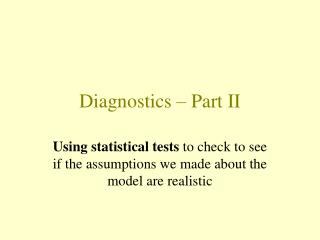Diagnostics – Part II

# Diagnostics – Part II - PowerPoint PPT Presentation

Diagnostics – Part II. Using statistical tests to check to see if the assumptions we made about the model are realistic. Diagnostic methods. Some simple (but subjective) plots. (Then) Some formal statistical tests. (Now). Simple linear regression model.I am the owner, or an agent authorized to act on behalf of the owner, of the copyrighted work described.
Download Presentation## Diagnostics – Part II

An Image/Link below is provided (as is) to download presentation

Download Policy: Content on the Website is provided to you AS IS for your information and personal use and may not be sold / licensed / shared on other websites without getting consent from its author.While downloading, if for some reason you are not able to download a presentation, the publisher may have deleted the file from their server.

- - - - - - - - - - - - - - - - - - - - - - - - - - E N D - - - - - - - - - - - - - - - - - - - - - - - - - -
Presentation Transcript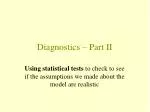### Diagnostics – Part II

Using statistical tests to check to see if the assumptions we made about the model are realistic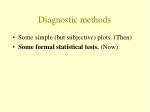Diagnostic methods
• Some simple (but subjective) plots.(Then)
• Some formal statistical tests. (Now)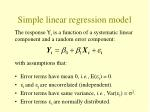Simple linear regression model

The response Yi is a function of a systematic linear component and a random error component:

with assumptions that:

• Error terms have mean 0, i.e., E(i) = 0.
• i and j are uncorrelated (independent).
• Error terms have same variance, i.e., Var(i) = 2.
• Error terms i are normally distributed.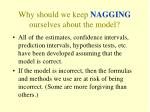Why should we keep NAGGING ourselves about the model?
• All of the estimates, confidence intervals, prediction intervals, hypothesis tests, etc. have been developed assuming that the model is correct.
• If the model is incorrect, then the formulas and methods we use are at risk of being incorrect. (Some are more forgiving than others.)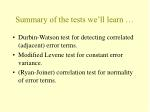Summary of the tests we’ll learn …
• Durbin-Watson test for detecting correlated (adjacent) error terms.
• Modified Levene test for constant error variance.
• (Ryan-Joiner) correlation test for normality of error terms.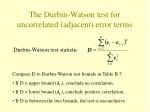Durbin-Watson test statistic

• Compare D to Durbin-Watson test bounds in Table B.7:
• If D > upper bound (dU), conclude no correlation.
• If D < lower bound (dL), conclude positive correlation.
• If D is between the two bounds, the test is inconclusive.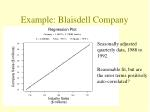Example: Blaisdell Company

Seasonally adjusted quarterly data, 1988 to 1992.

Reasonable fit, but are the error terms positively auto-correlated?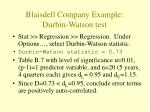Blaisdell Company Example: Durbin-Watson test
• Stat >> Regression >> Regression. Under Options…, select Durbin-Watson statistic.
• Durbin-Watson statistic = 0.73
• Table B.7 with level of significance α=0.01, (p-1)=1 predictor variable, and n=20 (5 years, 4 quarters each) gives dL= 0.95 and dU=1.15.
• Since D=0.73 < dL=0.95, conclude error terms are positively auto-correlated.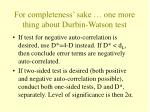For completeness’ sake … one more thing about Durbin-Watson test
• If test for negative auto-correlation is desired, use D*=4-D instead. If D* < dL, then conclude error terms are negatively auto-correlated.
• If two-sided test is desired (both positive and negative auto-correlation possible), conduct both one-sided tests, D and D*, separately. Level of significance is then 2α.Modified Levene Test for nonconstant error variance
• Divide the data set into two roughly equal-sized groups, based on the level of X.
• If the error variance is either increasing or decreasing with X, the absolute deviations of the residuals around their group median will be larger for one of the two groups.
• Two-sample t* to test whether mean of absolute deviations for one group differs significantly from mean of absolute deviations for second group.Modified Levene Test in Minitab
• Use Manip >> Code >> Numeric to numeric … to create a GROUP variable based on the values of X.
• Stat >> Regression >> Regression. Under Storage …, select residuals.
• Stat >> Basic statistics >> 2 Variances … Specify Samples (RESI1) and Subscripts (GROUP). Select OK. Look in session window for Levene P-value.Plutonium Alpha Example: Modified Levene’s Test

Levene's Test (any continuous distribution)

Test Statistic: 9.452

P-Value : 0.006

It is highly unlikely (P=0.006) that we’d get such an extreme Levene statistic (L=9.452) if the variances of the two groups were equal.

Reject the null hypothesis at the 0.01 level, and conclude that the error variances are not constant.• H0: Error terms are normally distributed vs. HA: Error terms are not normally distributed
• Stat >> Regression >> Regression. Under storage…, select residuals.
• Stat >> Basic statistics >> Normality Test. Select residuals (RESI1) and request Ryan-Joiner test. Select OK.